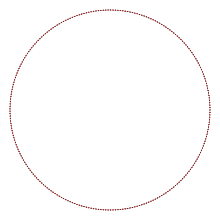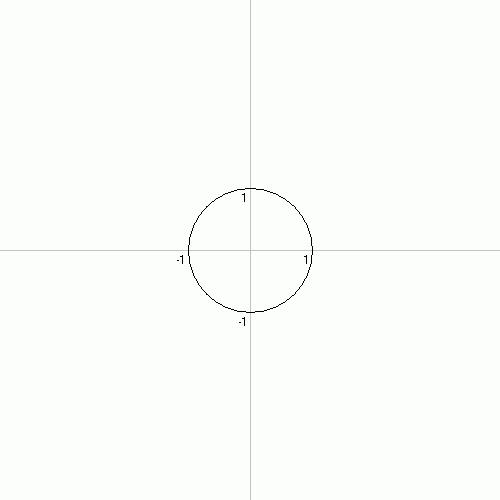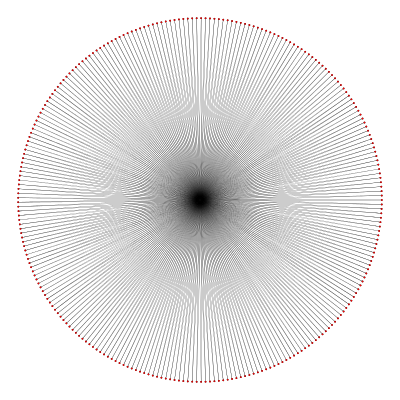# 257-gon

Regular 257-gonA regular 257-gon
Type Regular polygon
Edges and vertices 257
Schläfli symbol {257}
Coxeter diagramSymmetry group Dihedral (D257), order 2×257
Internal angle (degrees) ≈178.60°
Dual polygon Self
Properties Convex, cyclic, equilateral, isogonal, isotoxal

In geometry, a 257-gon (diacosipentacontaheptagon, diacosipentecontaheptagon) is a polygon with 257 sides. The sum of the interior angles of any non-self-intersecting 257-gon is 91800°.

## Regular 257-gon

The area of a regular 257-gon is (with t = edge length)A whole regular 257-gon is not visually discernible from a circle, and its perimeter differs from that of the circumscribed circle by about 24 parts per million.

### Construction

The regular 257-gon (one with all sides equal and all angles equal) is of interest for being a constructible polygon: that is, it can be constructed using a compass and an unmarked straightedge. This is because 257 is a Fermat prime, being of the form 22n + 1 (in this case n = 3). Thus, the valuesandare 128-degree algebraic numbers, and like all constructible numbers they can be written using square roots and no higher-order roots.

Although it was known to Gauss by 1801 that the regular 257-gon was constructible, the first explicit constructions of a regular 257-gon were given by Magnus Georg Paucker (1822) and Friedrich Julius Richelot (1832). Another method involves the use of 150 circles, 24 being Carlyle circles: this method is pictured below. One of these Carlyle circles solves the quadratic equation x2 + x  64 = 0.### Symmetry

The regular 257-gon has Dih257 symmetry, order 514. Since 257 is a prime number there is one subgroup with dihedral symmetry: Dih1, and 2 cyclic group symmetries: Z257, and Z1.

## 257-gram

A 257-gram is a 257-sided star polygon. As 257 is prime, there are 127 regular forms generated by Schläfli symbols {257/n} for all integers 2  n  128 as.

Below is a view of {257/128}, with 257 nearly radial edges, with its star vertex internal angles 180°/257 (~0.7°).## References

1. Magnus Georg Paucker (1822). "Das regelmäßige Zweyhundersiebenundfunfzig-Eck im Kreise.". Jahresverhandlungen der Kurländischen Gesellschaft für Literatur und Kunst (in German). 2: 188. Retrieved 8. December 2015.
2. Friedrich Julius Richelot (1832). "De resolutione algebraica aequationis x257 = 1, ...". Source: Journal für die reine und angewandte Mathematik (in Latin). 9: 1–26, 146–161, 209–230, 337–358. Retrieved 8. December 2015.
3. DeTemple, Duane W. (Feb 1991). "Carlyle circles and Lemoine simplicity of polygon constructions" (PDF). The American Mathematical Monthly. 98 (2): 97–108. doi:10.2307/2323939. Archived from the original (PDF) on 2015-12-21. Retrieved 6 November 2011.